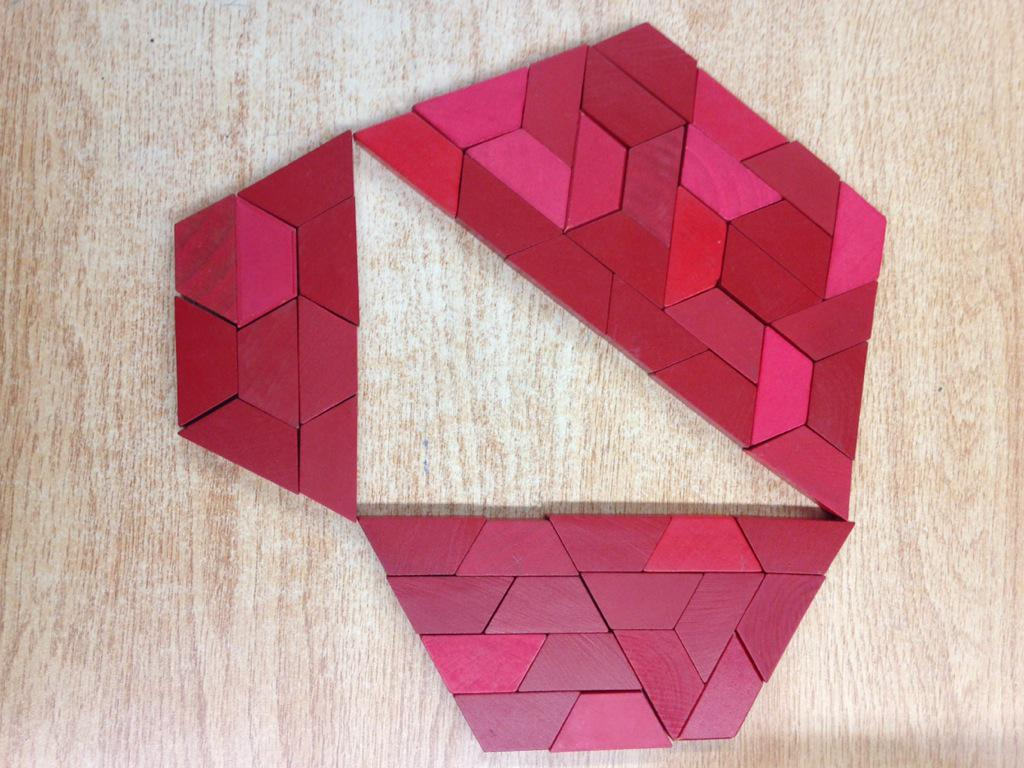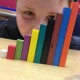# Is That Even A Problem???

Ask others what problem solving means with regard to mathematics.  Many will explain that a problem is when we put a real-world context to the mathematics being learned in class… others might explain a process of how we solve a problem (look at what you know and determine what you want to know, or some other set of strategies or a creative acronym that we have likely seen in school).  Sadly, much of what most others would point to as a problem is not really even a problem at all.

I think we all need to consider the real notion of what it means to problem solve…

George Polya shared this:…Thus, to have a problem means: to search consciously for some action appropriate to attain a clearly conceived, but not immediately attainable, aim. To solve a problem means to find such action. … Some degree of difficulty belongs to the very notion of a problem: where there is no difficulty, there is no problem.

What Polya is suggesting here is that if we show students how to do something, and then ask the students to practice that same thing in a context IT ISN’T A PROBLEM!!!  A problem in mathematics is like the DOING MATHEMATICS Tasks listed below.  Take a look at all 4 sections for a minute:My thoughts are simple… If a student can relatively quickly determine a course of action about how to get an answer, it isn’t a problem!   Even if the calculations are difficult or take a while.  The vast majority of what we call problems are actually just contextual practice of things we already knew.  Doing word problems IS NOT the same as problem solving!

On the other hand, if a student has to use REASONING skills, they are thinking, actively trying to figure something out, then and only then are they problem solving!!!

Marian Small has written a short article on her thoughts about problem solving:  Marian Small – Problem Solving

What are her main messages here?  Does this or Polya’s quote change your definition of a problem?

I’ve already written about What does Day 1 Look Like where I shared the importance of starting with problems.  So, why should we start with problem solving?  If we don’t start there, we aren’t likely ever doing any problem solving at all!

I also think that many hearing this might assume that this means we just hand students problems that they wouldn’t be successful with…  Ask everyone to attempt something that they wouldn’t know how to do.

Let’s look at an example:

Take a look at these two grade 8 expectations from Ontario curriculum:

• determine the Pythagorean relationship, through investigation using a variety of tools (e.g., dynamic geometry software; paper and scissors; geoboard) and strategies;
• solve problems involving right triangles geometrically

Many teachers look at these expectations, think to themselves, Pythagorean Theorem… I can teach that… followed by explicit teaching on a Smartboard (showing a video, modeling how the pythagorean theorem works, followed by some examples for the class to work on together…), then finally some problems that students have to answer in a textbook like this:This progression neither shows how we know students learn, nor does it even get students to be able to do what is asked!

Let’s take a step back and start to notice what the curriculum is saying more clearly:

• determine the Pythagorean relationship, through investigation using a variety of tools (e.g., dynamic geometry software; paper and scissors; geoboard) and strategies;
• solve problems involving right triangles geometrically, using the Pythagorean relationship;

When we pull apart the verbs and the tools/strategies from the content, we start to notice what the curriculum is telling us our students should actually be doing that day!  Remember… these expectations are what OUR STUDENTS should be doing… NOT US!!!

Above I have colored the verbs blue (These are the actions our students should be doing that day) and tools/strategies orange (specifically HOW our students should be accomplishing the verb).

Now let’s take a quick look at how this might actually play out in the classroom if we are starting with problems like our curriculum states:

Let’s start with the first expectation.  Our curriculum often includes the statement “determine through investigation,” yet it is overlooked far too often!  Students need to determine this themselves!  We need to assess students’ ability to “determine the Pythagorean relationship through investigation.”

This doesn’t mean we tell students what the theorem is, nor does it mean that we expect everyone to reinvent the theorem… so what does it mean???

Well, it could mean lots of things.  Here is one possible suggestion…Show the figure on the left.  Ask students the area of the blue square in the middle.  Possibly give a geoboard or paper and scissors for this task.  How might students come up with the area?  How many different ways might students accomplish this?

Share different approaches as a group.  (By the way, some calculate the whole shape and subtract the 4 corner triangles.  Others calculate the 4 black rectangles and divide by 2 then add 1 for the middle… others rearrange the shapes to make it make sense).

Now explore how the two pictures are similar / different.  What do you notice between the two pictures?

If the curriculum tells us to “determine through investigation” that is exactly the experience our students need to conceptualize the concept.  It is also what we need to assess.  This can’t be put on a test easily though, it needs to be observed!

That second expectation is quite interesting to me too.  At the beginning of this post we started talking about what is and isn’t a problem.  If our students have now understood what the Pythagorean Theorem is, how can we now make things problematic?

Showing a bunch of diagrams with missing hypotenuses or legs isn’t really problematic!

However, something like Dan Meyer’s Taco Cart would be!  If you haven’t seen the lesson, take a look:Oh… and by the way… problem solving isn’t always about answering a question… really, at it’s heart, problem solving is about making sense of things that we didn’t understand before… reasoning though things… noticing things we didn’t notice before… making conjectures and testing them out…  Problem solving is the process of LEARNING and DOING MATHEMATICS!

So I want to leave you with a problem for you to think about: what does this have to do with the Pythagorean Theorem?## 5 thoughts on “Is That Even A Problem???”

1.mikeollerton says:

‪#MTBoS I only have one strategy to support the differentiated states students are inevitably in. This is to offer starting point tasks which are accessible and ‘easily’ extendable. Once I have posed a problem, such as‬ using the numbers 1,2,3,4 the + and the = sign, make two 2-digit numbers and find all the different possible totals. Over this and ensuing lessons I will have many conversations with individual, pairs or small groups of students. Because they will be in mixed-attainment groups (no ‘tracking’ or ‘setting-by-‘ability’ in my classrooms) I shall have many in-the-moment unplanned for though not unexpected interactions; it is through these my approach to supporting students, all of whom will, collectively, be in a differentiated state.

If anyone wishes to see the subsequent extension ideas for my “1,2,3,4,+,=“ problem just email me: mikeollerton@btinternet.com

Like

1.Mark Chubb says:

I won hundred percent agree there’s not tracking or setting my ability. Offering different in the moment feedback or extensions is so much healthier than pre-assuming how much everyone could possibly learn.

Like